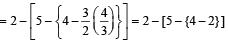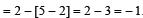# Olympiad Test: Rational Numbers -1

## 20 Questions MCQ Test Mathematical Olympiad Class 8 | Olympiad Test: Rational Numbers -1

Description
This mock test of Olympiad Test: Rational Numbers -1 for Class 8 helps you for every Class 8 entrance exam. This contains 20 Multiple Choice Questions for Class 8 Olympiad Test: Rational Numbers -1 (mcq) to study with solutions a complete question bank. The solved questions answers in this Olympiad Test: Rational Numbers -1 quiz give you a good mix of easy questions and tough questions. Class 8 students definitely take this Olympiad Test: Rational Numbers -1 exercise for a better result in the exam. You can find other Olympiad Test: Rational Numbers -1 extra questions, long questions & short questions for Class 8 on EduRev as well by searching above.
QUESTION: 1

### If 3/5 of a number exceeds its 2/7 by 44, then what is the number?

Solution:

Let the number be x.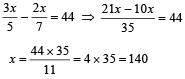QUESTION: 2

### A bus is moving at an average speed of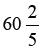km/hr. How much distance it will cover in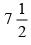hour?

Solution:

Distance = Speed × Time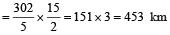QUESTION: 3

### The sum of two numbers is -4/3 If one of them is –5  then what is the other number?

Solution:
QUESTION: 4

In a school 5/8 of the students are boys. If the number of girls are 270. What is the number of boys in the school?

Solution:

No. of girls = 1 - 5/8 = 3/8
Let x be the total no. of students then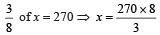x = 720
No. of boys = 720 - 270 = 450

QUESTION: 5

A cord of length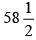m has been cut into 26 pieces of equal length. What is the length of each piece?

Solution:

Length of each piece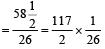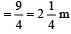QUESTION: 6

The product of two numbers is -16/35 . If one of the number is -15/14 what is the other number?

Solution:

Let the number be x.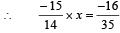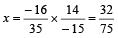QUESTION: 7

What should be subtracted from -5/3  to get 5/6 ?

Solution:
QUESTION: 8

What is additive inverse of -7/9?

Solution:

If x is the additive inverse then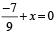x = 7/9

QUESTION: 9

The sum of two rational numbers is – 3. If one of the number is -10/3. What is the other number?

Solution: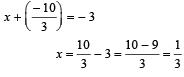QUESTION: 10

The cost of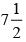metres of cloth is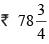.  What is the cost of one metre of cloth?

Solution:

Cost of one metre of cloth =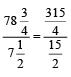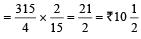QUESTION: 11

​By what number should -33/8 be divided to get -11/2 ?

Solution: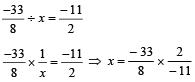=>  x= 3/4

QUESTION: 12

By what rational number should we multiplying -16/63 - to get -4/7.

Solution: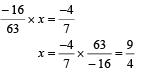QUESTION: 13

What number should be added to -7/8 to get 4/9 ?​

Solution: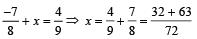x = 95/72

QUESTION: 14

What is reciprocal of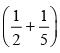?​

Solution:

We have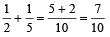∴    Reciprocal of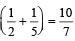QUESTION: 15

Which rational number is in between -2/3 and -1/4?

Solution:

Solution :- If a and b are two Rational numbers then a rational number between them is ( a + b )/2

Rational number between -2/3 and -1/4 = [ (-2/3) + (-1/4)]/2

= [ ( -8 - 3 )/12]/2

= [(-11)/12]/2

= -11/24

QUESTION: 16

What is the reciprocal of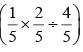?

Solution: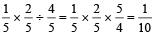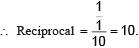QUESTION: 17

What should be added to -3/5 to get -1/3?

Solution: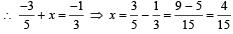QUESTION: 18

What is the additive inverse of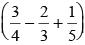?

Solution: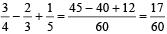Additive inverse of 17/60 is -17/60.

QUESTION: 19

What is the value of 3/4 ÷ 5/8 x 3/7 + 2/9 - 1/3 ?

Solution: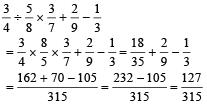QUESTION: 20

What is the value of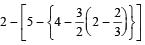Solution:

Here